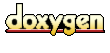# SimpleKLParameter::QueryModelParam Struct Reference

`#include <SimpleKLDocModel.hpp>`

List of all members.

## Public Attributes

double qryNoise
query noise coefficient

enum QueryUpdateMethod fbMethod
query model re-estimation/updating method

double fbCoeff
Q_new = (1-fbCoeff)*Q_old + fbCoeff*FBModel.

int fbTermCount
how many terms to use for the re-estimated query model

double fbPrTh
feedback query model term probability threshold (only terms with a higher prob. will be used

double fbPrSumTh
feedback query model prob. sum threshold (taking terms up to the point, where the accumulated prob. mass exceeds the threshold

double fbMixtureNoise
collection influence coefficient (e.g., in mixture model and divergence minimization methods)

int emIterations

## Member Data Documentation

 Q_new = (1-fbCoeff)*Q_old + fbCoeff*FBModel.

 query model re-estimation/updating method

 collection influence coefficient (e.g., in mixture model and divergence minimization methods)

 feedback query model prob. sum threshold (taking terms up to the point, where the accumulated prob. mass exceeds the threshold

 feedback query model term probability threshold (only terms with a higher prob. will be used

 how many terms to use for the re-estimated query model

 query noise coefficient

The documentation for this struct was generated from the following file:
Generated on Tue Jun 15 11:03:08 2010 for Lemur by1.3.4